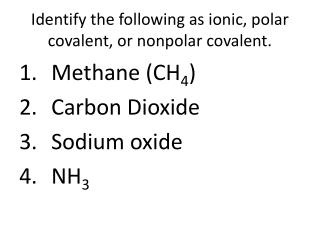Download PresentationIdentify the following as ionic, polar covalent, or nonpolar covalent.

# Identify the following as ionic, polar covalent, or nonpolar covalent.

Download Presentation## Identify the following as ionic, polar covalent, or nonpolar covalent.

- - - - - - - - - - - - - - - - - - - - - - - - - - - E N D - - - - - - - - - - - - - - - - - - - - - - - - - - -
##### Presentation Transcript

1. Identify the following as ionic, polar covalent, or nonpolar covalent. • Methane (CH4) • Carbon Dioxide • Sodium oxide • NH3

2. Reaction Spontaneity How can a fire start on its own? (without a spark)

3. Spontaneous Reaction • Occurs naturally and favors formation of products 4Fe(s) + 3O2(g)  2Fe2O3(s) ΔH = -1625 kJ CH4(g) + 2O2(g)  CO2(g) + 2H2O(l) ΔH = -891 kJ

4. Nonspontaneous Reaction Does not favor formation of products 2Fe2O3(s)  4Fe(s) + 3O2(g) ΔH = 1625 kJ CO2(g) + 2H2O(l)  CH4(g) + 2O2(g) ΔH = 891 kJ H2O(s)  H2O(l) ΔH = 6.01 kJ

5. Some endothermic reactions are SPONTANEOUS!

6. ENTROPY (S) • The measure of disorder or randomness of particles in a system LAW OF DISORDER Spontaneous processes always increase the entropy of a system

7. Law of Disorder

8. Changes in Entropy (ΔS) • Δssystem= Sproducts–Sreactants

9. 1. Changes in State

10. 2. The dissolving of a gas in a solvent always results in a decrease in entropy. CO2(g)  CO2(aq) Δssystem < 0

11. 3. Assuming no change in physical state, the entropy increases when: # of product particles > # of reactant particles. 2SO3(g)  2SO2(g) + O2(g) Δssystem > 0

12. 4. when a solid or a liquid dissolves to form a solution. NaCl(s)  Na+(aq) + Cl-(aq) Δssystem > 0

13. 5. increase in the temperature increase in entropy

14. Does entropy increase or decrease? CaCO3 (s)  CaO (s) + CO2 (g) NH3 (g) + HCl (g)  NH4Cl (s)

15. How ΔHsystem and ΔSsystemaffect reaction spontaneity

16. Gibb’s Free Energy!! ΔGsystem = ΔHsystem– TΔSsystem The energy that is available to do work (useful energy)

17. ΔGsystem and reaction spontaneity

18. Is the following reaction spontaneous? N2(g) + 3H2(g)  2NH3(g) ΔH°system= -91.8 kJΔS°system= -197 J/K ΔGsystem = ΔHsystem– TΔSsystem

19. For a process, ΔHsystem=145 kJ and ΔSsystem=322J/K. Is the reaction spontaneous at 382 K?